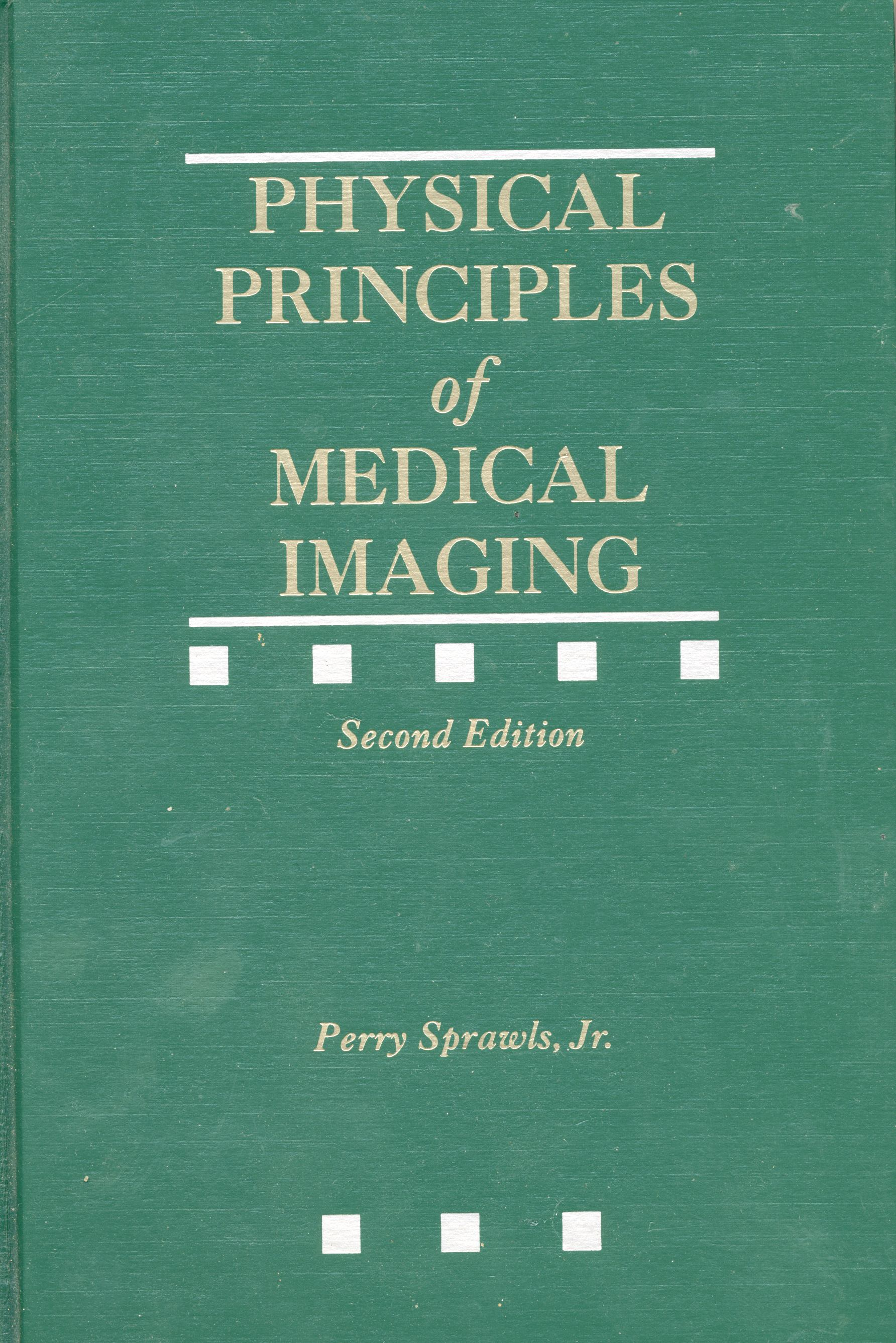Digital Image Characteristics
 Mind Map Learning Objectives Classroom Visuals Online Module Text Reference

 Outline and Learning Guide Introduction and Overview   Image Types Analog Images For human viewing Shades of gray Colors Digital Images For computer system processing, distribution, and storage Numbers   Digital Image Structure Pixels Concept Pixel values Matrix Pixel Bit Depth Concept Bits Number of bits Dynamic range Numerical Size Concept Significance Number of pixels Pixel bit depth   Digital Image Detail Concept Pixel Size Matrix size Image size (Field of view) The imaging modalities   Digital Image Contrast Concept Acquisition Dynamic Range Displayed Image Range Image Processing Windowing Concept Window level Window width   Image Compression Concept Why Compress Image storage Image transmission and distribution Levels of Compression Concept Lossless Lossy Compression Methods Concept JPEG Other   Summary

 Learning Objectives Give examples of typical analog images and describe their general characteristics. Explain why analog images are useful. Describe the general structure of a digital image in terms of pixels and bits. Briefly describe as many functions as possible that can be performed with digital medical images that are not possible with analog images. Explain the structural characteristic of a digital image that introduces blurring and limits visibility of detail. Explain and illustrate how pixel size is affected by the size of the image matrix. Explain and illustrate how pixel size is affected by the size of the imaged area (field of view). Explain and illustrate how computers write numbers and the concept of bits. Illustrate how the range of values that can be written is related to the number of bits being used. Briefly compare the methods used by both humans and computers to write numbers and some general advantages and disadvantages of each. Describe and illustrate the concept of bit depth of a pixel. Identify and describe the characteristic of an image that is affected by the bit depth. Illustrate and describe how bit depth is represented by both bits and bytes. Identify the factors that determine the numerical size of a digital image. When you are given the appropriate factor values, calculate the numerical size of an image in: bits, bytes, kilobytes, and megabytes. Explain how the numerical size of a digital image relates to image quality. Explain why the numerical size of a digital image is a limiting factor in some applications. Briefly describe the concept of digital image compression. Explain what is meant by "lossless" compression. Explain what is meant by "lossy" compression and what must be considered in clinical applications.Text Reference Physical Principles of Medical Imaging, 2nd Ed Chapter 22. Medical Physics Publishing,  http://www.medicalphysics.org